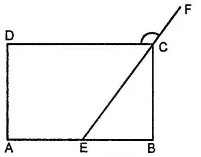The following figures GUNS and RUNS are parallelograms. Find x and y. (Lengths are in cm)Asked by Aaryan | 1 year ago |  143

##### Solution :-

(i)We know that the lengths of opposite sides of a parallelogram are equal to each other.

GU = SN

3y - 1 = 26

3y = 27

y = 9

SG = NU

3x = 18

x = 6

Hence, the measures of x and y are 6 cm and 9 cm respectively.

(ii)We know that the diagonals of a parallelogram bisect each other.

y + 7 = 20

y = 13 x + y = 16

x + 13 = 16

x = 3

Hence, the measures of x and y are 3 cm and 13 cm respectively.

Answered by Sakshi | 1 year ago

### Related Questions

#### In the given figure, PQRS is a kite. Find the values of x and y.

In the given figure, PQRS is a kite. Find the values of x and y.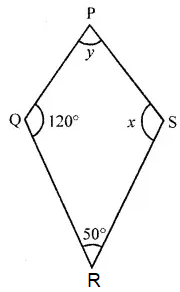#### In the given isosceles trapezium ABCD, ∠C = 102°. Find all the remaining angles of the trapezium.

In the given isosceles trapezium ABCD, ∠C = 102°. Find all the remaining angles of the trapezium.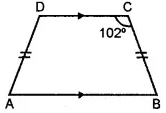#### In the given figure, ABCD is a rhombus and ∠ABD = 50°. Find :

In the given figure, ABCD is a rhombus and ∠ABD = 50°. Find :

(i) ∠CAB

(ii) ∠BCD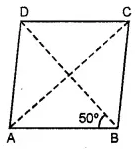#### In the given figure, ABCD is a rectangle and diagonals intersect at O. If ∠AOB = 118°, find

In the given figure, ABCD is a rectangle and diagonals intersect at O. If ∠AOB = 118°, find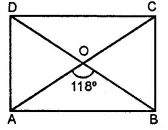(i) ∠ABO

(iii) ∠OCB